##### Actions

A general strategy for computing linear and cyclic convolutions by applying the polynomial version of the Chinese remainder theorem [a1]. For polynomialsand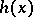of degree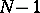and, respectively, the linear convolution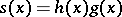has degree, where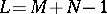. For any polynomial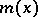of degree, the linear convolution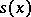can be computed by computing the productmodulo, i.e.,The Chinese remainder theorem permits this computation to be localized. Choose a factorizationinto relatively prime factors. The Winograd small convolution algorithm proceeds by first computing the reduced polynomials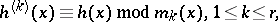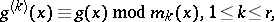followed by the local computationsand is completed by combining these local computations using the formula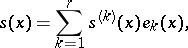where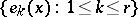is a complete system of idempotents corresponding to the initial factorization of.

S. Winograd [a2] expanded on this general strategy by developing bilinear algorithms for computing the product of polynomials modulo a polynomial. Within this general strategy, these bilinear algorithms permit one to use small efficient algorithms as building blocks for larger-size algorithms [a3].